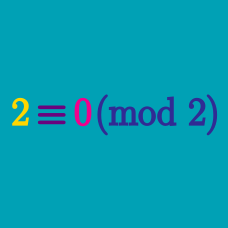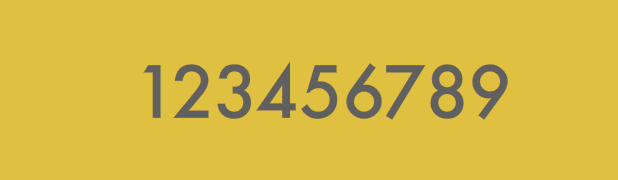Number Theory

# Modular Arithmetic Operations: Level 2 Challenges

Find the last digit when

$\color{#3D99F6}1^{\color{#3D99F6}1} + \color{#20A900}2^{\color{#20A900}2} + \color{#69047E}3^{ \color{#69047E}3} + \cdots + \color{#D61F06}9^{ \color{#D61F06}9} + \color{#BA33D6}{10}^{\color{#BA33D6}{10}}$

is written out as an integer.

What is $5 ^ {-1} \pmod{17} ?$

Hint: Remember that inverses multiply to 1.Given that $2^{29}$ is a nine-digit number with distinct digits, determine (without evaluating $2^{29}$) which one of the ten digits is missing.

What is the sum of all possible primes $p$ such that $p^2+8$ is also a prime?

How many natural numbers $n$ exist such that the following are all primes?

$3n-4 \qquad 4n-5 \qquad 5n-3$

×